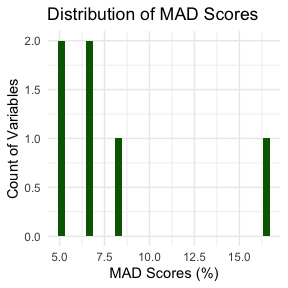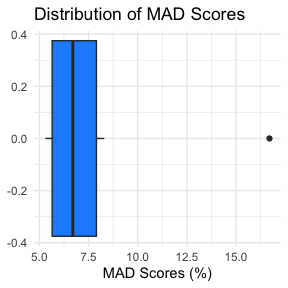## Introductory Remarks

A central question in any imputation effort is whether the imputed values you came up with are any good or not.

Though several metrics for evaluating imputations exist, a common one is mean absolute differences (MAD scores) between original and imputed values. This gives a very practical look at how close or far off the imputations were from the original data.

MAD scores are computed at the variable level through the calculation of mean absolute differences between the original distribution of cases and the imputed version of those same cases. Practically, MAD shows aggregate error when imputing over individual variables. Lower percentages mean less error/differences compared to higher percentages, which mean greater overall error/differences across all variables between original and imputed data sets.

For example, suppose you had a nominal variable with three potential values A, B, and C. The distribution of the variable across each category was A = 40%, B = 30%, and C = 30% when considering only complete cases. Then, after imputing this variable, you observed the distribution A = 43%, B = 28%, and C = 29%. We would calculate the mean absolute difference as $$(|40-43| + |30-28| + |30-29|) / 3 = 2$$, or 2% average difference between the original and imputed versions of the same variable. The logic is easily scaled up to accommodate high dimensional data spaces, with identical interpretation making it a very intuitive and helpful evaluative metric for imputations tasks. Note: the bigger the data space, the slower the computation.

Let’s see this in action in the following section via the mad() function from the latest release of hdImpute.

First, load the library along with the tidyverse library for some additional helpers in setting up the sample data space.

library(hdImpute)
library(tidyverse)

Next, set up the data and introduce missingness completely at random (MCAR) via the prodNA() function from the missForest package. Take a look at the synthetic data with missingness introduced.

d <- data.frame(X1 = c(1:6),
X2 = c(rep("A", 3),
rep("B", 3)),
X3 = c(3:8),
X4 = c(5:10),
X5 = c(rep("A", 3),
rep("B", 3)),
X6 = c(6,3,9,4,4,6))

set.seed(1234)

data <- missForest::prodNA(d, noNA = 0.30) %>%
as_tibble()

data
#> # A tibble: 6 × 6
#>      X1 X2       X3    X4 X5       X6
#>   <int> <chr> <int> <int> <chr> <dbl>
#> 1     1 <NA>      3     5 A         6
#> 2    NA A         4     6 A         3
#> 3     3 <NA>      5     7 A         9
#> 4    NA B        NA    NA <NA>      4
#> 5    NA B         7     9 B        NA
#> 6    NA B         8    10 B         6

Note: This is a tiny sample set, but hopefully the usage is clear enough.

First, impute this simple data set via hdImpute():

imputed = hdImpute(data = data, batch = 2)
#>
#> Missing value imputation by random forests
#>
#>   Variables to impute:       X1
#>   Variables used to impute:  X1
#> iter 1:  .
#>
#> Missing value imputation by random forests
#>
#>   Variables to impute:       X3, X2
#>   Variables used to impute:  X3, X2
#> iter 1:  ..
#> iter 2:  ..
#>
#> Missing value imputation by random forests
#>
#>   Variables to impute:       X4, X5
#>   Variables used to impute:  X4, X5
#> iter 1:  ..
#> iter 2:  ..
#>
#> Missing value imputation by random forests
#>
#>   Variables to impute:       X6
#>   Variables used to impute:  X6
#> iter 1:  .

Now, we have an imputed versions of the original data space with no more missingness.

imputed
#> # A tibble: 6 × 6
#>      X1 X2       X3    X4 X5       X6
#>   <int> <chr> <int> <int> <chr> <dbl>
#> 1     1 B         3     5 A         6
#> 2     1 A         4     6 A         3
#> 3     3 B         5     7 A         9
#> 4     1 B         5     7 A         4
#> 5     1 B         7     9 B         9
#> 6     3 B         8    10 B         6

But how good is this at capturing the original distribution of the data (pre-imputation)? Let’s find out by computing MAD scores for each variable via mad()

mad(original = data,
imputed = imputed,
round = 1)
#> # A tibble: 6 × 2
#>   <chr> <dbl>
#> 1 X1     16.7
#> 2 X2      8.3
#> 3 X3      5.3
#> 4 X4      5.3
#> 5 X5      6.7
#> 6 X6      6.7

We can see we did best on X3 and X4 with scores at 5.3% mean difference for each, and worst on X1 with a score of 16.7% mean difference. Importantly, precisely what defines “best” or “worst” MAD is entirely project-dependent. Users should interpret results with care.

By default, the function returns a tibble. This can easily be stored in an object for later use:

mad_scores <- mad(original = data,
imputed = imputed,
round = 1)

Now, with our mad_scores as a tidy tibble, we can continuing working with it to, e.g., visualize the distribution of error across this full data space with only a few lines of code (remember: lower MAD is better, meaning fewer average differences in the distribution of imputations compared to the original data).

First, a histogram:

mad_scores %>%
geom_histogram(fill = "dark green") +
labs(x = "MAD Scores (%)", y = "Count of Variables", title = "Distribution of MAD Scores") +
theme_minimal() +
theme(legend.position = "none")Or a boxplot:

mad_scores %>%
geom_boxplot(fill = "dodgerblue") +
labs(x = "MAD Scores (%)", title = "Distribution of MAD Scores") +
theme_minimal() +
theme(legend.position = "none")## Concluding Remarks

This software is being actively developed, with many more features to come. Wide engagement with it and collaboration is welcomed! Here’s a sampling of how to contribute:

• Submit an issue reporting a bug, requesting a feature enhancement, etc.

• Suggest changes directly via a pull request

• Reach out directly with ideas if you’re uneasy with public interaction

Thanks for using the tool. I hope its useful.